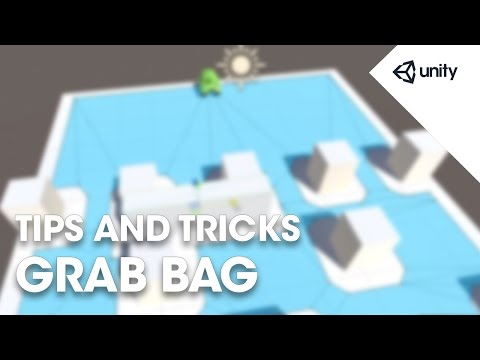# Unity Tips and Tricks Grab Bag

#### 难度: 中级

Who doesn't like #unitytips? In this session we will look at a bunch of different tips and tricks that you may not have known about. Topics include how to: Visualizing in the scene view with editor extensions, modularizing assets, and using particles for performance gain. We are literally cramming as much stuff into an hour session as we can, so who knows how much we'll cover! Let's find out! Trainer: Mike Geig## Unity Tips and Tricks Grab Bag

Scripts for the navigation editor extensions

### Code snippet

``````using UnityEngine;

public class CharacterNavigation : MonoBehaviour
{
public Transform target;

NavMeshAgent agent;

void Awake()
{
agent = GetComponent<NavMeshAgent>();
}

void Update ()
{
agent.SetDestination(target.position);
agent.Stop();
}
}``````

### Code snippet

``````using UnityEngine;
using UnityEditor;

public class CharacterNavigationEditor : Editor
{
static bool visualizePath = false;

public override void OnInspectorGUI()
{
DrawDefaultInspector();

visualizePath = EditorGUILayout.Toggle("Visualize Path", visualizePath);
}

[DrawGizmo(GizmoType.Selected | GizmoType.NonSelected)]
static void DrawLinesForNavAgent(NavMeshAgent agent, GizmoType gizmoType)
{
if (!visualizePath || agent.path == null)
return;

Gizmos.color = Color.red;
Vector3[] points = agent.path.corners;
for (int i = 0; i < points.Length - 1; i++)
{
Gizmos.DrawLine(points[i], points[i + 1]);
}
}
}``````

Scripts for the particle optimizations

### Code snippet

``````using UnityEngine;
using System.Collections;

public class EmitterScript : MonoBehaviour
{
public float rate = 20f;
public int maxCoins = 100;
public GameObject coin;

Rigidbody2D[] coins;
int index;
float amntPerUpdate;

void Start ()
{
coins = new Rigidbody2D[maxCoins];
for (int i = 0; i < maxCoins; i++)
{
coins[i] = (Instantiate(coin) as GameObject).GetComponent<Rigidbody2D>();
coins[i].gameObject.SetActive(false);
}

amntPerUpdate = rate * .02f;
index = 0;
}

void FixedUpdate()
{
for (int i = 0; i < amntPerUpdate; i++)
{
coins[index].gameObject.SetActive(false);
coins[index].transform.position = transform.position;
coins[index].transform.rotation = Quaternion.identity;
coins[index].gameObject.SetActive(true);

Vector3 velocity = new Vector3(Random.Range(-2f, 2f), 10f, 0f);
coins[index].velocity = velocity;

if (++index >= maxCoins)
index = 0;
}
}
}``````

### Code snippet

``````using UnityEngine;

public class ParticleCoinScript : MonoBehaviour
{
public ParticleSystem coinSystem;
public Transform playerPosition;
public float floorHeight = 0f;

int maxParticles;
ParticleSystem.Particle[] coins;

void Start()
{
maxParticles = coinSystem.maxParticles;
coins = new ParticleSystem.Particle[maxParticles];
}

void Update()
{
coinSystem.GetParticles(coins);

for (int i = 0; i < coins.Length; i++)
{
//Bounce
if (coins[i].position.y < floorHeight)
{
if (coins[i].velocity.y < 0)
coins[i].velocity = new Vector3(coins[i].velocity.x, 5, coins[i].velocity.z);
else
coins[i].position = new Vector3(coins[i].position.x, floorHeight, coins[i].position.z);
}

//Collect by player
float distance = Vector3.Distance(coins[i].position, playerPosition.position);
if (Mathf.Abs(distance) <= 1.5f)
}

coinSystem.SetParticles(coins, maxParticles);
}
}``````

#### 社区资源#### Tips

1. Unity Tips & Tricks
2. Unity Tips & Tricks 2
3. Unity Tips & Tricks 3
4. Unity Tips & Tricks 4
5. Unity Tips and Tricks Grab Bag